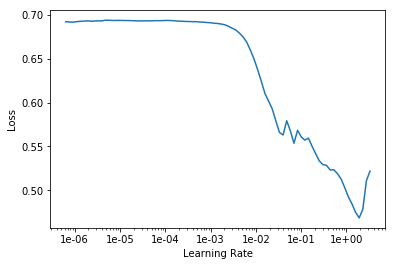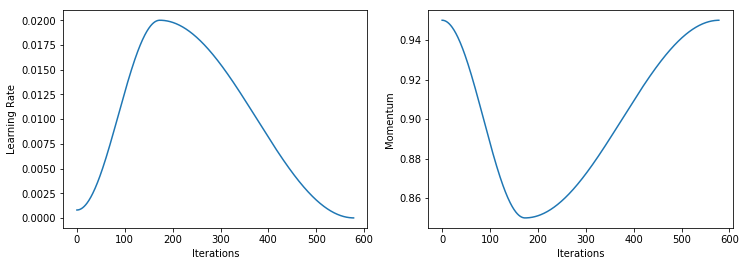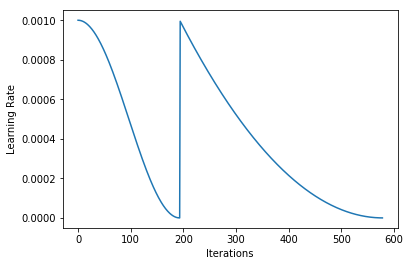Callbacks implemented in the fastai library

## List of callbacks¶

fastai's training loop is highly extensible, with a rich callback system. See the `callback` docs if you're interested in writing your own callback. See below for a list of callbacks that are provided with fastai, grouped by the module they're defined in.

Every callback that is passed to `Learner` with the `callback_fns` parameter will be automatically stored as an attribute. The attribute name is snake-cased, so for instance `ActivationStats` will appear as `learn.activation_stats` (assuming your object is named `learn`).

## `Callback`¶

This sub-package contains more sophisticated callbacks that each are in their own module. They are (click the link for more details):

### `LRFinder`¶

Use Leslie Smith's learning rate finder to find a good learning rate for training your model. Let's see an example of use on the MNIST dataset with a simple CNN.

```path = untar_data(URLs.MNIST_SAMPLE)
data = ImageDataBunch.from_folder(path)
def simple_learner(): return Learner(data, simple_cnn((3,16,16,2)), metrics=[accuracy])
learn = simple_learner()
```

The fastai librairy already has a Learner method called `lr_find` that uses `LRFinder` to plot the loss as a function of the learning rate

```learn.lr_find()
```
```LR Finder is complete, type {learner_name}.recorder.plot() to see the graph.
```
```learn.recorder.plot()
```In this example, a learning rate around 2e-2 seems like the right fit.

```lr = 2e-2
```

### `OneCycleScheduler`¶

Train with Leslie Smith's 1cycle annealing method. Let's train our simple learner using the one cycle policy.

```learn.fit_one_cycle(3, lr)
```
Total time: 00:07

epoch train_loss valid_loss accuracy time
0 0.109439 0.059349 0.980864 00:02
1 0.039582 0.023152 0.992149 00:02
2 0.019009 0.021239 0.991659 00:02

The learning rate and the momentum were changed during the epochs as follows (more info on the dedicated documentation page).

```learn.recorder.plot_lr(show_moms=True)
```### `MixUpCallback`¶

Data augmentation using the method from mixup: Beyond Empirical Risk Minimization. It is very simple to add mixup in fastai :

```learn = Learner(data, simple_cnn((3, 16, 16, 2)), metrics=[accuracy]).mixup()
```

### `CSVLogger`¶

Log the results of training in a csv file. Simply pass the CSVLogger callback to the Learner.

```learn = Learner(data, simple_cnn((3, 16, 16, 2)), metrics=[accuracy, error_rate], callback_fns=[CSVLogger])
```
```learn.fit(3)
```
Total time: 00:07

epoch train_loss valid_loss accuracy error_rate time
0 0.127259 0.098069 0.969578 0.030422 00:02
1 0.084601 0.068024 0.974975 0.025025 00:02
2 0.055074 0.047266 0.983317 0.016683 00:02

You can then read the csv.

```learn.csv_logger.read_logged_file()
```
epoch train_loss valid_loss accuracy error_rate
0 0 0.127259 0.098069 0.969578 0.030422
1 1 0.084601 0.068024 0.974975 0.025025
2 2 0.055074 0.047266 0.983317 0.016683

### `GeneralScheduler`¶

Create your own multi-stage annealing schemes with a convenient API. To illustrate, let's implement a 2 phase schedule.

```def fit_odd_shedule(learn, lr):
n = len(learn.data.train_dl)
phases = [TrainingPhase(n).schedule_hp('lr', lr, anneal=annealing_cos),
TrainingPhase(n*2).schedule_hp('lr', lr, anneal=annealing_poly(2))]
sched = GeneralScheduler(learn, phases)
learn.callbacks.append(sched)
total_epochs = 3
learn.fit(total_epochs)
```
```learn = Learner(data, simple_cnn((3,16,16,2)), metrics=accuracy)
fit_odd_shedule(learn, 1e-3)
```
Total time: 00:07

epoch train_loss valid_loss accuracy time
0 0.176607 0.157229 0.946025 00:02
1 0.140903 0.133690 0.954367 00:02
2 0.130910 0.131156 0.956820 00:02
```learn.recorder.plot_lr()
```### `MixedPrecision`¶

Use fp16 to take advantage of tensor cores on recent NVIDIA GPUs for a 200% or more speedup.

### `HookCallback`¶

Convenient wrapper for registering and automatically deregistering PyTorch hooks. Also contains pre-defined hook callback: `ActivationStats`.

### `RNNTrainer`¶

Callback taking care of all the tweaks to train an RNN.

### `TerminateOnNaNCallback`¶

Stop training if the loss reaches NaN.

### `EarlyStoppingCallback`¶

Stop training if a given metric/validation loss doesn't improve.

### `SaveModelCallback`¶

Save the model at every epoch, or the best model for a given metric/validation loss.

```learn = Learner(data, simple_cnn((3,16,16,2)), metrics=accuracy)
learn.fit_one_cycle(3,1e-4, callbacks=[SaveModelCallback(learn, every='epoch', monitor='accuracy')])
```
Total time: 00:07

epoch train_loss valid_loss accuracy time
0 0.679189 0.646599 0.804220 00:02
1 0.527475 0.497290 0.908243 00:02
2 0.464756 0.462471 0.917076 00:02
```!ls ~/.fastai/data/mnist_sample/models
```
```best.pth	 bestmodel_2.pth  model_1.pth  model_4.pth    stage-1.pth
bestmodel_0.pth  bestmodel_3.pth  model_2.pth  model_5.pth    tmp.pth
bestmodel_1.pth  model_0.pth	  model_3.pth  one_epoch.pth  trained_model.pth
```

### `ReduceLROnPlateauCallback`¶

Reduce the learning rate each time a given metric/validation loss doesn't improve by a certain factor.

### `PeakMemMetric`¶

GPU and general RAM profiling callback

### `StopAfterNBatches`¶

Stop training after n batches of the first epoch.

### `LearnerTensorboardWriter`¶

Broadly useful callback for Learners that writes to Tensorboard. Writes model histograms, losses/metrics, embedding projector and gradient stats.

## `train` and `basic_train`¶

### `Recorder`¶

Track per-batch and per-epoch smoothed losses and metrics.

### `ShowGraph`¶

Dynamically display a learning chart during training.

### `BnFreeze`¶

Freeze batchnorm layer moving average statistics for non-trainable layers.